# Venn diagrams in Python

What is Venn diagram?

• Sets of shapes especially circles to represent the logical relationships among two or more variables or datasets
• Venn diagrams are useful to identify the shared and unique elements belonging to each variable or datasets For example, in genomics experiments Venn diagrams are useful to identify the shared and unique genes among different conditions

How to create a Venn diagrams using Python?

``````# We will use `bioinfokit` for plotting 2 and 3-way Venn diagrams.
git clone https://github.com/reneshbedre/bioinfokit.git
cd bioinfokit
python setup.py install
``````

Plot 2 and 3-way Venn diagrams using bioinfokit

``````# you can use interactive python console, jupyter or python code
# I am using interactive python console (Python 3.6)
>>>from bioinfokit import visuz
# 3-way Venn
>>>visuz.venn(vennset=(1,1,1,1,1,1,1))
# note: order the set in (100,010,110,001,101,011,111) format

# 2-way venn
>>>visuz.venn(vennset=(1,1,1))
# note: order the set in (111,100,010,001,110,101,011) format
``````

Generated Venn plots by above code,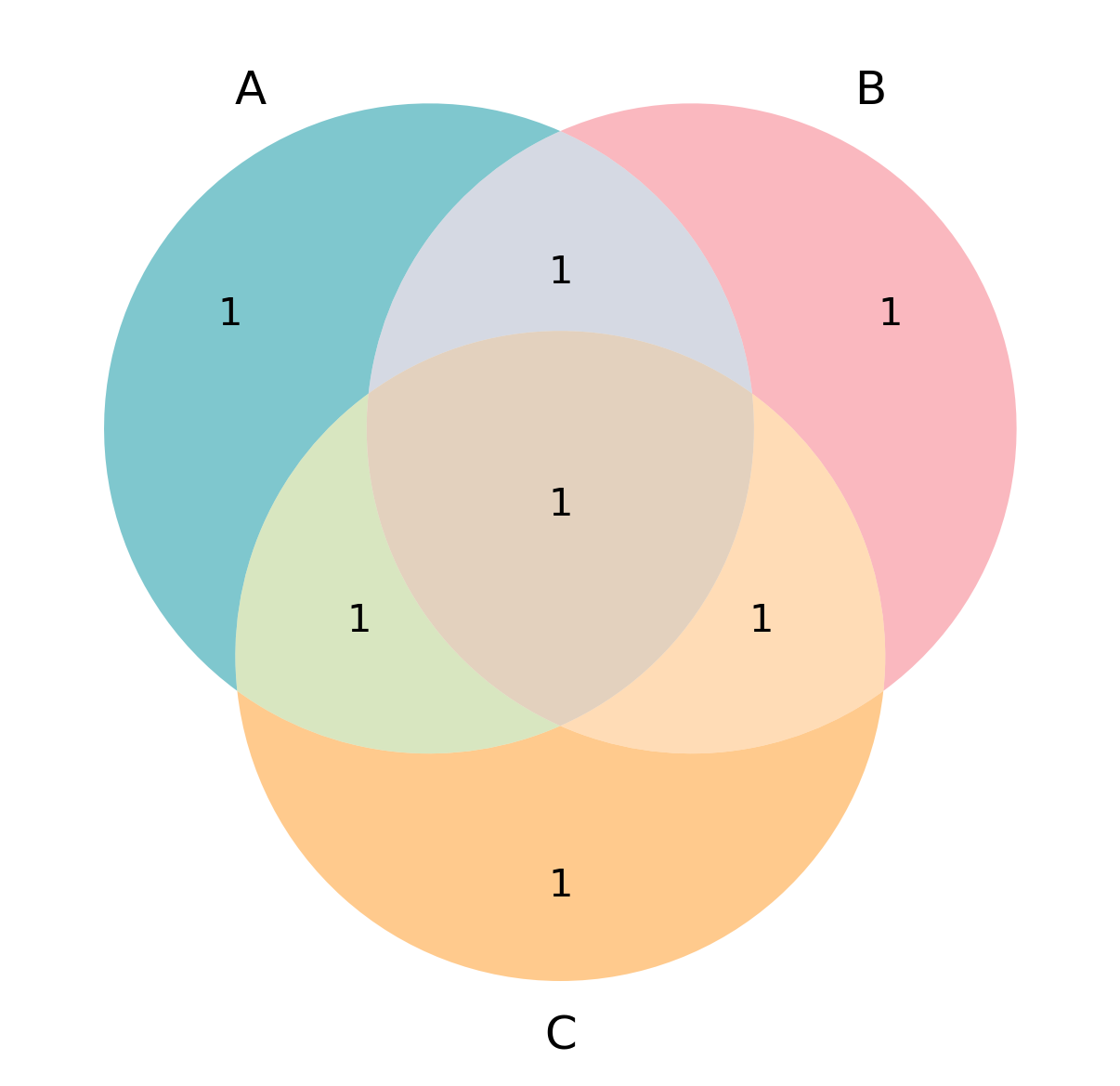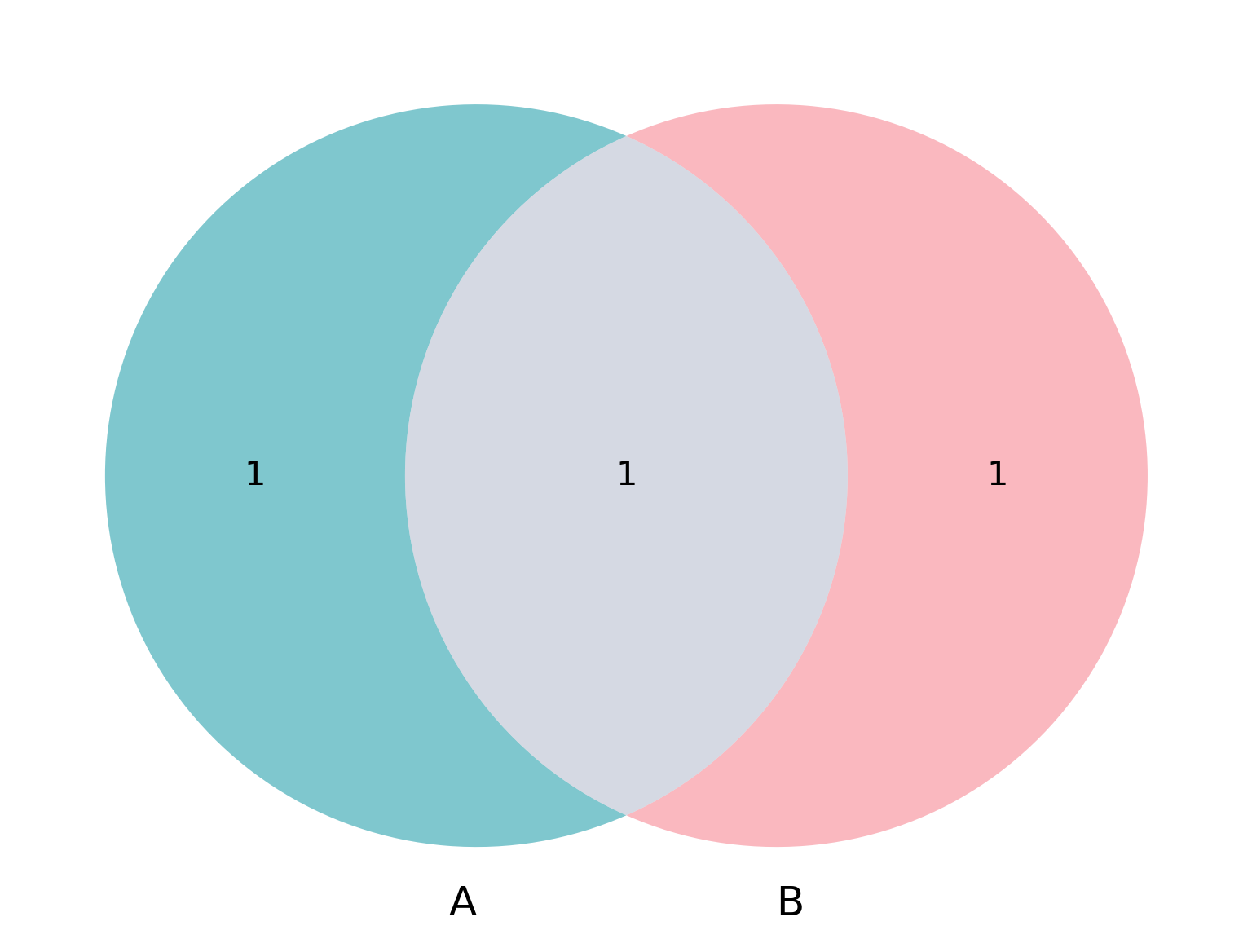With changes in default parameters for sets, and color, and labels

``````>>>visuz.venn(vennset=(300,600,402,470,200,230,408), vennlabel=("X", "Y", "Z"))
# note: order the set in (100,010,110,001,101,011,111) format

# change default color
# check for more colors at https://www.webucator.com/blog/2015/03/python-color-constants-module/
>>>visuz.venn(vennset=(300,600,402,470,200,230,408), vennlabel=("X", "Y", "Z"), venncolor=('#ff6347', '#008000', '#FF00FF'))
``````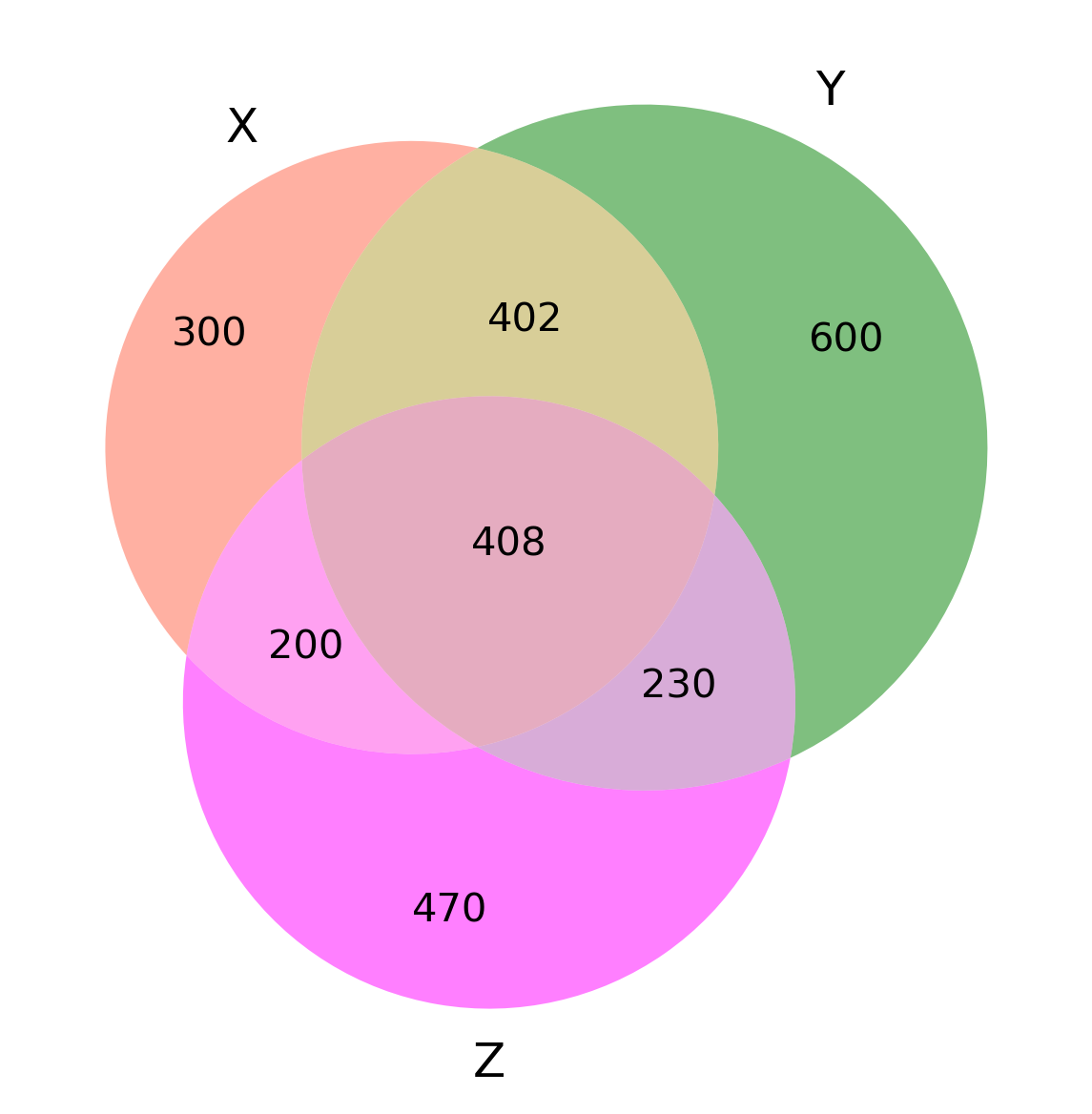With changes in default parameters for transparency

``````>>>visuz.venn(vennset=(300,600,402,470,200,230,408), vennlabel=("X", "Y", "Z"), vennalpha=0.9)
# note: order the set in (100,010,110,001,101,011,111) format

# 2-way venn
>>>visuz.venn(vennset=(300,600,402), vennlabel=("X", "Y"), venncolor=('#ff6347', '#008000'), vennalpha=0.9)
# note: order the set in (10,01,11) format
``````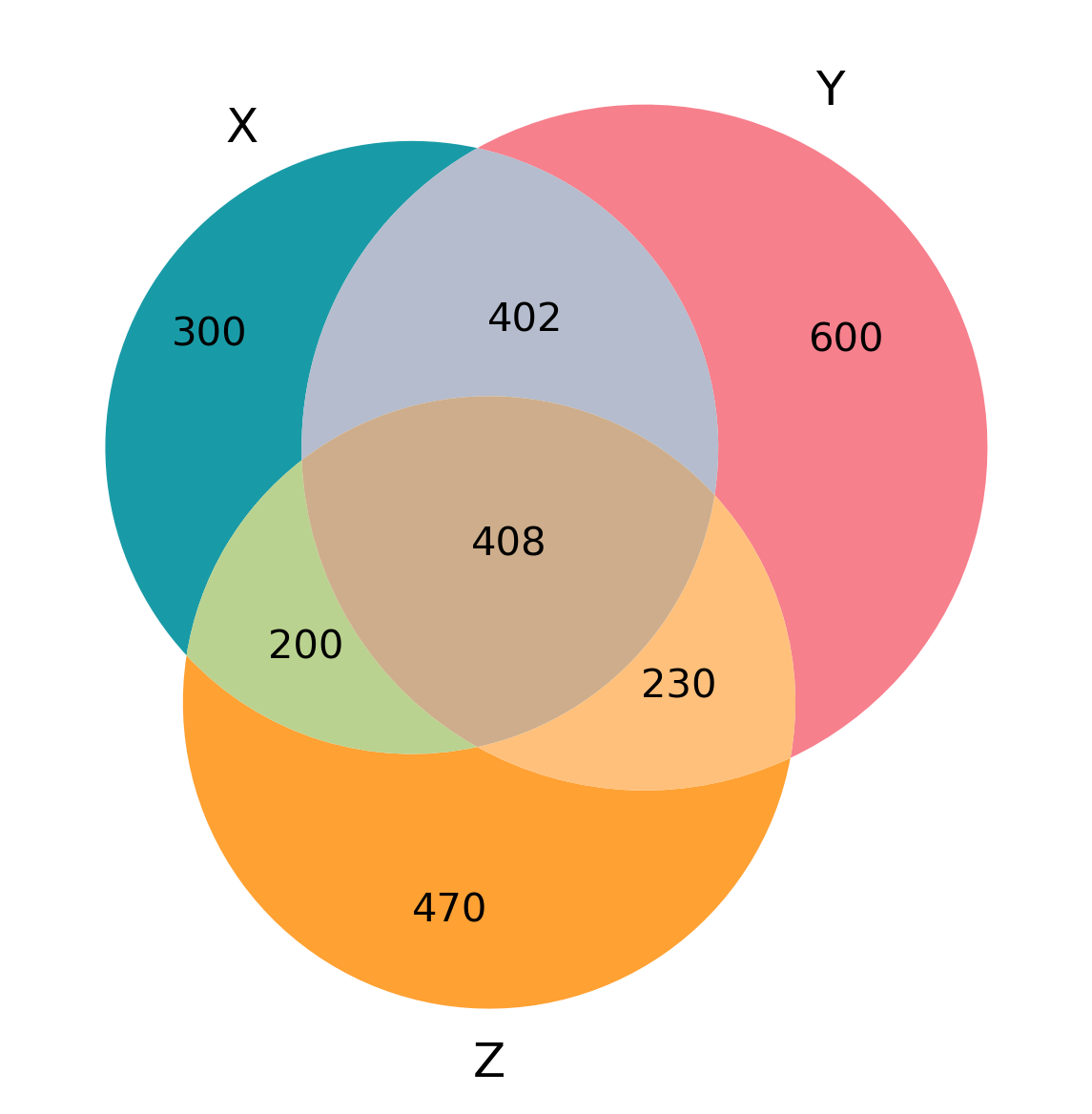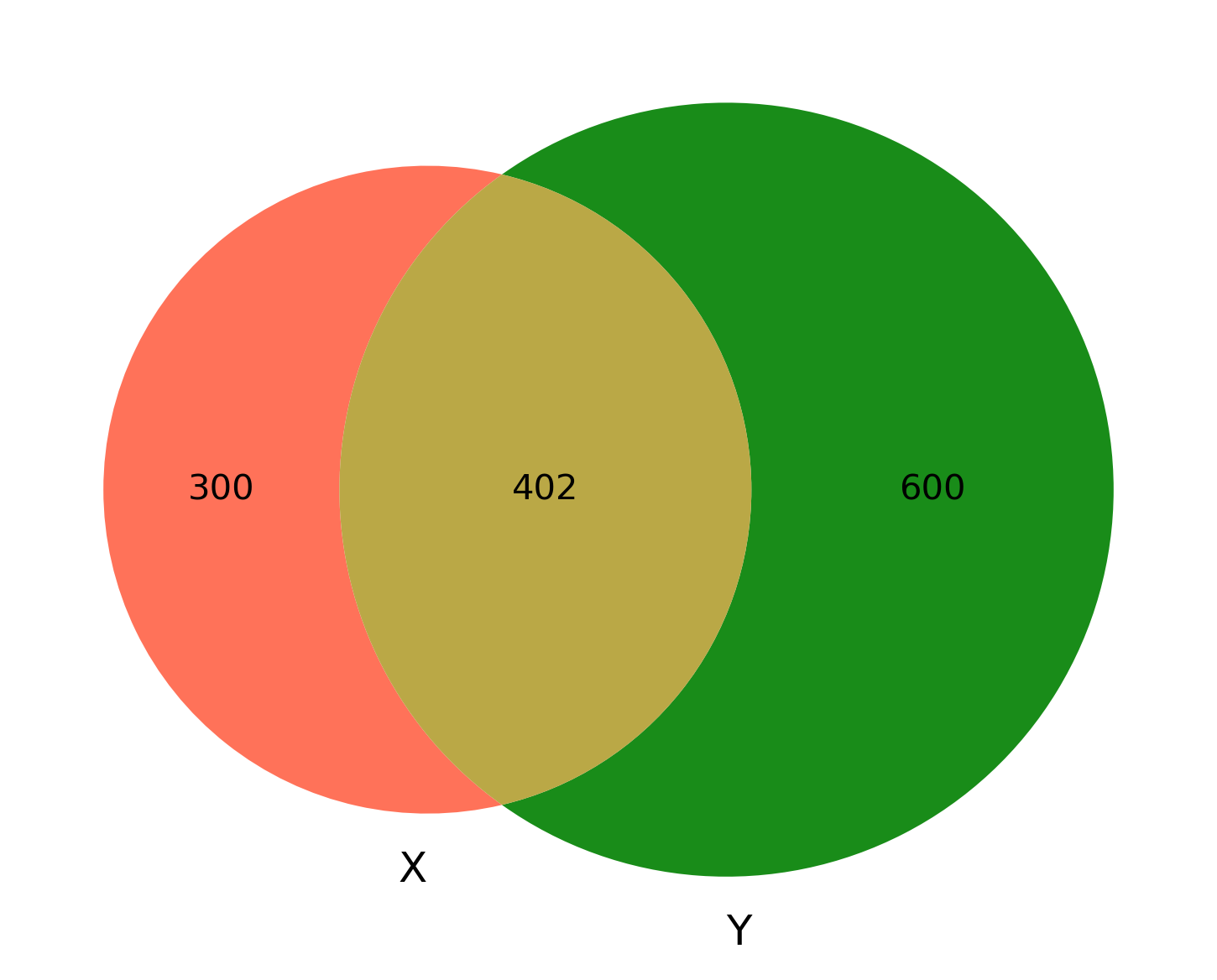• https://github.com/konstantint/matplotlib-venn

How to cite?
Renesh Bedre.(2020, July 29). reneshbedre/bioinfokit: Bioinformatics data analysis and visualization toolkit (Version v0.9). Zenodo. http://doi.org/10.5281/zenodo.3965241

If you have any questions, comments or recommendations, please email me at reneshbe@gmail.com

Last updated: December 13, 2019